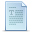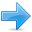### C++ Language

• #### Standard library

In C++, two different functions can have the same name if their parameters are different; either because they have a different number of parameters, or because any of their parameters are of a different type. For example:

 ``````// overloading functions #include using namespace std; int operate (int a, int b) { return (a*b); } double operate (double a, double b) { return (a/b); } int main () { int x=5,y=2; double n=5.0,m=2.0; cout << operate (x,y) << '\n'; cout << operate (n,m) << '\n'; return 0; }`````` ```10 2.5```

In this example, there are two functions called `operate`, but one of them has two parameters of type `int`, while the other has them of type `double`. The compiler knows which one to call in each case by examining the types passed as arguments when the function is called. If it is called with two `int` arguments, it calls to the function that has two `int` parameters, and if it is called with two `double`s, it calls the one with two `double`s.

In this example, both functions have quite different behaviors, the `int` version multiplies its arguments, while the `double` version divides them. This is generally not a good idea. Two functions with the same name are generally expected to have -at least- a similar behavior, but this example demonstrates that is entirely possible for them not to. Two overloaded functions (i.e., two functions with the same name) have entirely different definitions; they are, for all purposes, different functions, that only happen to have the same name.

Note that a function cannot be overloaded only by its return type. At least one of its parameters must have a different type.

### Function templates

Overloaded functions may have the same definition. For example:

 ``````// overloaded functions #include using namespace std; int sum (int a, int b) { return a+b; } double sum (double a, double b) { return a+b; } int main () { cout << sum (10,20) << '\n'; cout << sum (1.0,1.5) << '\n'; return 0; }`````` ```30 2.5```

Here, `sum` is overloaded with different parameter types, but with the exact same body.

The function `sum` could be overloaded for a lot of types, and it could make sense for all of them to have the same body. For cases such as this, C++ has the ability to define functions with generic types, known as function templates. Defining a function template follows the same syntax as a regular function, except that it is preceded by the `template` keyword and a series of template parameters enclosed in angle-brackets <>:

`template <template-parameters> function-declaration`
The template parameters are a series of parameters separated by commas. These parameters can be generic template types by specifying either the `class` or `typename` keyword followed by an identifier. This identifier can then be used in the function declaration as if it was a regular type. For example, a generic `sum` function could be defined as:

 ``12345`` ``````template SomeType sum (SomeType a, SomeType b) { return a+b; }``````

It makes no difference whether the generic type is specified with keyword `class` or keyword `typename` in the template argument list (they are 100% synonyms in template declarations).

In the code above, declaring `SomeType` (a generic type within the template parameters enclosed in angle-brackets) allows `SomeType` to be used anywhere in the function definition, just as any other type; it can be used as the type for parameters, as return type, or to declare new variables of this type. In all cases, it represents a generic type that will be determined on the moment the template is instantiated.

Instantiating a template is applying the template to create a function using particular types or values for its template parameters. This is done by calling the function template, with the same syntax as calling a regular function, but specifying the template arguments enclosed in angle brackets:

`name <template-arguments> (function-arguments)`
For example, the `sum` function template defined above can be called with:

 ``1`` ``x = sum(10,20);``

The function `sum<int>` is just one of the possible instantiations of function template `sum`. In this case, by using `int` as template argument in the call, the compiler automatically instantiates a version of `sum` where each occurrence of `SomeType` is replaced by `int`, as if it was defined as:

 ``1234`` ``````int sum (int a, int b) { return a+b; }``````

Let's see an actual example:

 ``````// function template #include using namespace std; template T sum (T a, T b) { T result; result = a + b; return result; } int main () { int i=5, j=6, k; double f=2.0, g=0.5, h; k=sum(i,j); h=sum(f,g); cout << k << '\n'; cout << h << '\n'; return 0; }`````` ```11 2.5```

In this case, we have used `T` as the template parameter name, instead of `SomeType`. It makes no difference, and `T` is actually a quite common template parameter name for generic types.

In the example above, we used the function template `sum` twice. The first time with arguments of type `int`, and the second one with arguments of type `double`. The compiler has instantiated and then called each time the appropriate version of the function.

Note also how `T` is also used to declare a local variable of that (generic) type within `sum`:

 ``1`` ``T result;``

Therefore, result will be a variable of the same type as the parameters `a` and `b`, and as the type returned by the function.
In this specific case where the generic type `T` is used as a parameter for `sum`, the compiler is even able to deduce the data type automatically without having to explicitly specify it within angle brackets. Therefore, instead of explicitly specifying the template arguments with:

 ``12`` ``````k = sum (i,j); h = sum (f,g);``````

It is possible to instead simply write:
 ``12`` ``````k = sum (i,j); h = sum (f,g);``````

without the type enclosed in angle brackets. Naturally, for that, the type shall be unambiguous. If `sum` is called with arguments of different types, the compiler may not be able to deduce the type of `T` automatically.

Templates are a powerful and versatile feature. They can have multiple template parameters, and the function can still use regular non-templated types. For example:

 ``````// function templates #include using namespace std; template bool are_equal (T a, U b) { return (a==b); } int main () { if (are_equal(10,10.0)) cout << "x and y are equal\n"; else cout << "x and y are not equal\n"; return 0; }`````` `x and y are equal`

Note that this example uses automatic template parameter deduction in the call to `are_equal`:

 ``1`` ``are_equal(10,10.0)``

Is equivalent to:

 ``1`` ``are_equal(10,10.0)``

There is no ambiguity possible because numerical literals are always of a specific type: Unless otherwise specified with a suffix, integer literals always produce values of type `int`, and floating-point literals always produce values of type `double`. Therefore `10` has always type `int` and `10.0` has always type `double`.

### Non-type template arguments

The template parameters can not only include types introduced by `class` or `typename`, but can also include expressions of a particular type:

 ``````// template arguments #include using namespace std; template T fixed_multiply (T val) { return val * N; } int main() { std::cout << fixed_multiply(10) << '\n'; std::cout << fixed_multiply(10) << '\n'; }`````` ```20 30```

The second argument of the `fixed_multiply` function template is of type `int`. It just looks like a regular function parameter, and can actually be used just like one.

But there exists a major difference: the value of template parameters is determined on compile-time to generate a different instantiation of the function `fixed_multiply`, and thus the value of that argument is never passed during runtime: The two calls to `fixed_multiply` in `main` essentially call two versions of the function: one that always multiplies by two, and one that always multiplies by three. For that same reason, the second template argument needs to be a constant expression (it cannot be passed a variable).Previous:FunctionsIndexNext:Name visibility